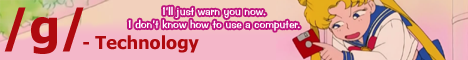[a / b / c / d / e / f / g / gif / h / hr / k / m / o / p / r / s / t / u / v / vg / vr / w / wg] [i / ic] [r9k / s4s / vip / qa] [cm / hm / lgbt / y] [3 / aco / adv / an / asp / bant / biz / cgl / ck / co / diy / fa / fit / gd / hc / his / int / jp / lit / mlp / mu / n / news / out / po / pol / qst / sci / soc / sp / tg / toy / trv / tv / vp / wsg / wsr / x] [Settings] [Search] [Home]
Board
/sci/ - Science & MathThis question is bizarre to me, I don't know what I'm proving. From a is congruent to 7 mod(9), that just means a is the set {7, 16, 25, 34, 43, 52...}, right? What am I supposed to fucking do with this?
>>
>>10800258
No, it does not mean a is the set {7, 16, ...}, it means a IS IN that set. It even says that a and b are integers, not sets (they're elements of Z, not subsets). I never studied number theory so I don't actually know exactly how to prove your result, but I imagine that, for (a), you simply show that the number you got satisfies both conditions.
>>
>>10800289
But saying a is congruent to 7 mod(9) would imply that a could be 7, 16, 25, 34, 43, 52 and so on, wouldn't it? You're right, it's not the whole set of them, but it is one integer within that set isn't it? I know that x absolutely has to be 1 but I don't know how to go about "proving" that. If I just arbitrarily pick a value for a, it becomes simple but I'm not sure I'm allowed to do that.
>>
>>10800298
No, you don't arbitrarily pick a number, you show that for any number you pick for a, having x=1 (and y=1 as well, right?) will mean that it also satisfies a =_ x( mod 3). You do this by showing that every element of 7( mod 9) also belongs to 1( mod 3). Same for y and b.
>>
>>10800301
More formally, you would say

Let a =_ 7( mod 9).
Then (a-7)/9 is an integer.
Thus [(a-7)/9] * 3 = (a-7)/3 = (a-1)/3 - 6/3 = (a-1)/3 - 2 is similarly an integer (since, given x, y, z are integers, (xy + z) is also an integer).
Since (a-1)/3 is an integer, this means that a =_ 1( mod 3).
Hence for any a =_ 7( mod 9) ==> a =_ 1( mod 3). QED.
>>
>>10800258
a=7+9n
a=x=1 (mod 3)
x=1

b=1+6m
b=y=1 (mod 3)
y=1

a^2 + 2ab = 1 + 2 = 0 (mod 3)
>>
>>10800289
wrong, a is an equivalence class you fucking retard.
>>
>>10800423
So I was right the first time?
>>
>>10800423
It isn't though, a is simply IN the equivalence class.

In the example provided, clearly 37 isn't an equivalence class. However, it does belong to the equivalence class  of mod 10.

Source: https://en.wikipedia.org/wiki/Congruence_relation

>>10800439
No, you're not, a isn't a class, it's an integer.
>>
>>10800353
Why does a equal 1?
>>
>>10800473
I think, in lines 2 and 5, = is supposed to be the triple equals sign, i.e. congruent equivalence (which I will denote as =_). So what he meant is that:
a =_ x =_ 1 (mod 3)
b =_ y =_ 1 (mod 3)

So he didn't say that a=x=1, he said that a is congruent modulo 3 to x, which is congruent to 1 modulo 3.

Delete Post: [File Only] Style: